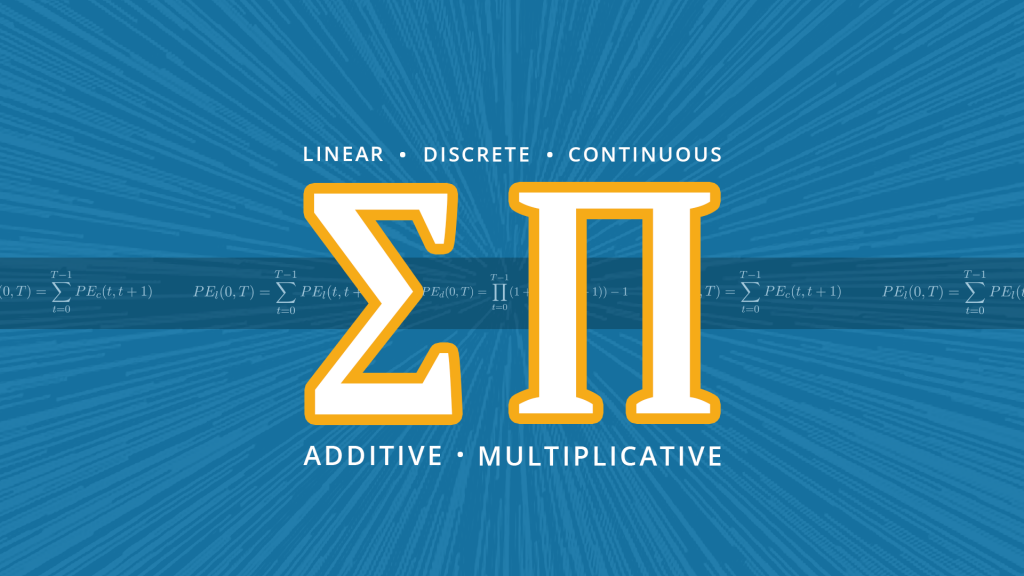In this week’s article I wanted to dig a little deeper into the financial maths side of statistical measures. I thought it would be helpful to explain and prove some very important properties of linear, discrete and continuous returns. In common literature only discrete and continuous returns are analyzed as those are the only ones most commonly applied in market practice. However, as many of our clients ask questions about linear returns we wanted to include this use case in today’s article.

In our last blog post we explained how performance figures are correctly calculated. It is an important consideration that performance figures vary due to the different assumptions on how interest is paid and reinvested. If we were to take one example, a security being priced now at 130 while we initially invested at a price of 100 has a discrete effective return ofand a continuous effective return of. So the performance figures are different, nevertheless, we still earned 30 units.

In the remainder of this blog post we wanted to show how the respective performance figures behave in a multi-period setting and how single-period returns can be aggregated to an overall multi-period return. We will also prove that discrete returns are not additive, but multiplicative whereas linear and continuous returns are additive. With this knowledge it will be easier to understand our upcoming articles about calculating more advanced metrics and risk statistics.

Proofing additivity for returns assumes a multi-period setting, thus we calculate the linear effective performance usingwiththe price at time. For this we can prove using induction that linear returns are additive:

To prove:Basis:Inductive step:### Discrete returns are not additive, but multiplicative:

For discrete returns the following holds:In the end of every period interest is paid and reinvested. First we see that discrete returns are not additive:But discrete returns are multiplicative. This can be proofed again using induction:

To prove:Basis:Inductive step:For continuous returns interest is paid continuously and not only at discrete points in time. We have:You can proof that continuous returns are additive using induction for a last time:

To prove:Basis:Inductive step:WEBINAR